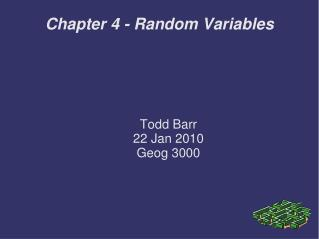# Chapter 4 - Random Variables - PowerPoint PPT PresentationDownload PresentationChapter 4 - Random Variables

Chapter 4 - Random Variables
Download Presentation## Chapter 4 - Random Variables

- - - - - - - - - - - - - - - - - - - - - - - - - - - E N D - - - - - - - - - - - - - - - - - - - - - - - - - - -
##### Presentation Transcript

1. Todd Barr 22 Jan 2010 Geog 3000 Chapter 4 - Random Variables

2. Overview • Discuss the types of Random Variables • Discrete • Continuous • Discuss the Probability Density Function • What can you do with Random Variables

3. Random Variables • Is usually represented by an upper case X • is a variable whose potential values are all the possible numeric outcomes of an experiment • Two types that will be discussed in this presentation are Discrete and Continuous

4. Discrete Random Variables • Discrete Random Variables are whole numbers (0,1,3,19.....1,000,006) • They can be obtained by counting • Normally, they are a finite number of values, but can be infinite if you are willing to count that high.

5. Discrete Probability Distribution • Discrete Probability Distributions are a description of probabilistic problem where the values that are observed are contained within predefined values • As with all discrete numbers the predefined values must be countable • They must be mutually exclusive • They must also be exhaustive

6. Discrete Probability Distro Example • The classic fair coin example is the best way to demonstrate Discrete Probability

7. Classic Coin Example • Experiment: Toss 2 Coins and Count the Numbers of Tails

8. Classic Coin Continued • Histogram of our tosses

9. Classic Coin Toss Summary • Its easy to see from the Histogram on the previous slide the area that each of the results occupy • If we repeat this test 1000 times, there is a strong probability that our results will resemble the previous Histogram but with some standard variance • For more on the classic coin toss and Discrete Random Variables please go see the Educator video at http://www.youtube.com/watch?v=T6eoHAjdAfM

10. Its all Greek to Me • Mean and Variables of Random Variables, symbology • μ (Mu) is the symbol for population mean • σ is the symbol for standard deviation • s or x-bar are the symbols for data

11. Mean of Probability Distribution • The Mean of Probability Distribution is a weighted average of all the possible values within an experiment • It assists in controlling for outliers and its important to determining Expected Value

12. Expected Value • Within the discrete experiment, an expected value is the probability weighted sums of all the potential values • Is symbolized by E[X]

13. Variance • Variance is the expected value from the Mean. • The Standard Deviation is the Square Root of the Variance

14. Continuous Random Variables • Continuous Random Variables are defined by ranges on a number line, between 0 and 1 • This leads to an infinite range of probabilities • Each value is equally likely to occur within this range

15. Continuous Random Variables • Since it would nearly impossible to predict the precise value of a CRV, you must include it within a range. • Such as, you know you are not going to get precisely 2” of rain, but you could put a range at Pr(1.99≤x≤2.10) • This will give you a range of probability on the bell curve, or the Probability Density Function

16. Probability Density Function • The Probability Density of a Continuous Random Variable is the area under the curve between points a and n in your formula • In the above Bell Curve the Probability Density formula would be Pr(.57≤x≤.70) • For a more detailed explanation please see the Khan Academy at www.KhanAcademy.org

17. Adding Random Variables • By knowing the Mean and Variance of of a Random Variable you can use this to help predict outcomes of other Random Variables • Once you create a new Random Variable, you can use the other Random Variables within your experiment to develop a more robust test • As long as the Random Variables are independent this process is simple • If the Random Variables are Dependent, then this process becomes more difficult